Stratonovich integral

Letbe continuous semi-martingales (cf. Semi-martingale) defined on a filtered probability space. The Stratonovich integral ofwith respect toon the interval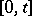is defined as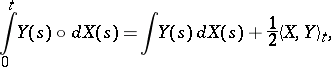(a1)

where the integral on the right-hand side is the Itô stochastic integral and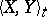denotes the quadratic cross-variation process ofand. There is no universally agreed notation for the integral, but the above is the most common (see [a1], [a2], for example). It is also known as the Fisk, Fisk–Stratonovich or symmetrized stochastic integral, the latter in view of the property that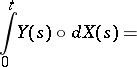(a2)wheredenotes a partition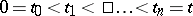,, and the limit is in probability. Indeed, this property was the original definition of the integral, [a3], [a4]. As an immediate application of (a2) one sees that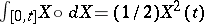, and this points to the main feature of the Stratonovich integral, namely that the Itô formula expressed in terms of Stratonovich integrals coincides with the "ordinary" (Newton–Leibniz) formula. Let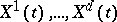be continuous semi-martingales, defining, and let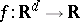be a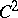-function. The Itô formula is(a3)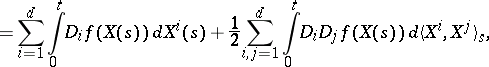where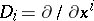. If one writes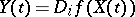, then for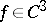it is readily verified that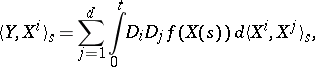(a4)

and hence (a3) becomes simply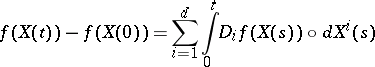(a5)

— the formula of "ordinary calculus" . Equations (a4), (a5) remain valid for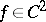[a2], Thm. V20.

At first sight the Stratonovich integral appears to be simply a notational trick to obtain (a5). However, it plays an important role in several areas of stochastic analysis, including:

a) Approximation of stochastic differential equations, [a1], Sect. V1.7. Let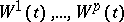be independent Brownian motions (cf. Brownian motion) and consider the stochastic differential equation(a6)

where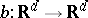and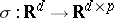are bounded functions with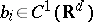and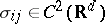. Written in terms of Stratonovich integrals this becomes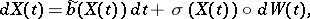(a7)

where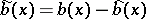and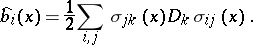Now, letbe a piecewise-linear approximation to the Brownian path, i.e.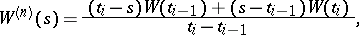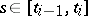, for a partition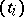ofas above, and consider the approximating sequence of random ordinary differential equations(a8)

where the dot denotes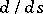. ThenThus, the approximation scheme (a8) derives naturally from the Stratonovich equation (a7), and not from the Itô equation (a6). In general, the limit obtained depends on the specific approximation to the Brownian path chosen, unless thecolumns of, considered as vector fields (cf. Vector field), commute, i.e.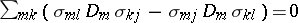,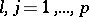, in which case the limit (a6) or (a7) is obtained for any "reasonable" approximation to the Brownian path; in particular, this is true when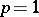. Questions of this sort were first investigated by E. Wong and M. Zakai [a5], and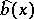is sometimes known as the Wong–Zakai correction term.

b) Support of diffusion processes, [a1], Sect. V1.8, [a6]. Consider the solution of (a6) or (a7) starting from a fixed point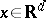. It defines a measure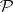on the sample space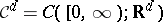. Let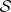be the support of this measure, i.e. the smallest closed set in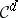with-measure. Letbe the set of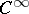-functions in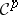, and, for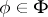, let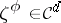be the solution of the ordinary differential equation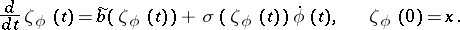Then. Thusis just the closure of the set of "outputs"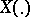of equation (a7) when the "input"is replaced by smooth functions. As in a), the Stratonovich formulation is required to preserve consistency between systems with "smooth" and "Brownian" inputs.

c) Diffusion processes on manifolds. Letbe a-manifold (cf. Manifold), letbe smooth vector fields on(cf. Vector field on a manifold), and fix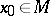. Then there is a unique-valued process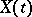such that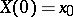and for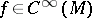,(a9)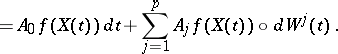It is essential to use the Stratonovich integral here; the same equation written with Itô integrals fails to provide a coordinate-independent description, since the rules of Itô calculus conflict with the ordinary rules of calculus relating different coordinate systems. It is immediate from (a9) thatand hence that the Itô form of (a9) is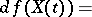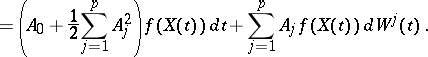It follows readily thatis a diffusion process with differential generatorA detailed account of the Stratonovich integral and its properties is contained in [a2], Sect. V.5, including an extension of the definition to possibly discontinuous semi-martingales.